In :
# Set up imports and matplotlib options
%pylab inline
import numpy as np
import matplotlib.pyplot as plt
import quantities as pq
from matplotlib import rc
rc('font',**{'family':'sans-serif',
'sans-serif': 'Helvetica, Arial',
'weight': 'medium',
'size': 18})
rc('text', usetex=False)
rc('lines', linewidth=3)

Welcome to pylab, a matplotlib-based Python environment [backend: module://IPython.zmq.pylab.backend_inline].
For more information, type 'help(pylab)'.

# Scientific computing with Python¶

Python is widely used for translating scientific ideas to code. Excellent packages are available to do the types of things that scientists do on a daily basis.

• NumPy makes it easy to work with large chunks of data (i.e., vectors and matrices)
• SciPy provides packages for doing common scientific computing tasks
• Matplotlib creates beautiful plots quickly and with fine-grained control

In this set of examples, I'm going to try to convince you that Quantities should also be in the list of packages scientists use on a daily basis.

The following question comes from Physics: Principles and Problems by Paul Zitzewitz.

A hockey puck, mass 0.115 kg, moving at 35.0 m/s, strikes a rubber octopus thrown on the ice by a fan. The octopus has a mass of 0.265 kg. The puck and octopus slide off together. Find their velocity.

The solution to this is based on the conservation of momentum: the momentum of moving puck and the stationary octopus before the collision is equal to their combined momentum after the collision.

\begin{aligned} m_p v_p + m_o v_o &= (m_p + m_o) v_{po} \\\\ v_{po} &= \frac{m_p v_p + m_o v_o}{m_p + m_o} \\\\ v_{po} &= \frac{0.115 \times 35 + 0.265 \times 0.0}{0.115 + 0.265} \end{aligned}

Let's use Python to do this computation.

In :
mp = 0.115
vp = 35.0
mo = 0.265
vo = 0.0

v = (mp * vp + mo * vo) / (mp + mo)
print v

10.5921052632

### Data exploration¶

To explore the situation being described in this question, we can try changing some of these values and redoing the computation.

An obvious thing to change would be the puck speed. How does the velocity of the combined puck and octopus (pucktopus) change when the puck has a higher initial speed?

In :
vp = 40.0
v = (mp * vp + mo * vo) / (mp + mo)
print v

12.1052631579

NumPy and Matplotlib allows us to do this exploration much faster.

Rather than changing vp and recalculting v, we can make vp into a vector of many numbers using np.arange.

In :
vp = np.arange(51.)
v = (mp * vp + mo * vo) / (mp + mo)
print v

[  0.           0.30263158   0.60526316   0.90789474   1.21052632
1.51315789   1.81578947   2.11842105   2.42105263   2.72368421
3.02631579   3.32894737   3.63157895   3.93421053   4.23684211
4.53947368   4.84210526   5.14473684   5.44736842   5.75         6.05263158
6.35526316   6.65789474   6.96052632   7.26315789   7.56578947
7.86842105   8.17105263   8.47368421   8.77631579   9.07894737
9.38157895   9.68421053   9.98684211  10.28947368  10.59210526
10.89473684  11.19736842  11.5         11.80263158  12.10526316
12.40789474  12.71052632  13.01315789  13.31578947  13.61842105
13.92105263  14.22368421  14.52631579  14.82894737  15.13157895]

Note that we didn't have to change the update for v at all; NumPy just does the calculation with all of the vp values!

Outputting the numbers is a bit too much to process visually, so let's instead plot this data on a graph.

In :
plt.plot(vp, v)

Out:
[<matplotlib.lines.Line2D at 0x10aded1d0>]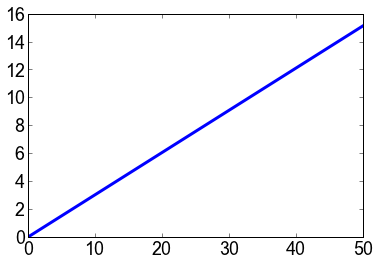We can easily see that there is a linear relationship between the velocity of the puck before the collision and the velocity of the pucktopus after the collision.

What if, instead, we varied the mass of the puck?

In :
vp = 35.0
mp = np.arange(0., 2.05, 0.05)
v = (mp * vp + mo * vo) / (mp + mo)
plt.plot(mp, v)

Out:
[<matplotlib.lines.Line2D at 0x10372e210>]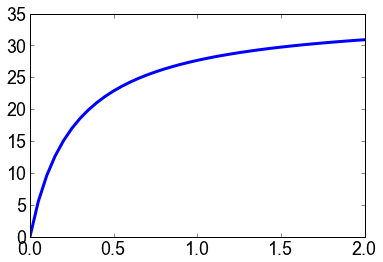This looks logarithmic, which is much more interesting! As we increase the mass of the puck, the pucktopus velocity quickly increases, but starts to plateau as we reach 1.0 kg.

## Units matter¶

However, if we handed in these scripts to our high school Physics teacher, he or she might be impressed by the Python scripts, but will ultimately mark us down for not including our units. Recall the original problem:

A hockey puck, mass 0.115 kg, moving at 35.0 m/s, strikes a rubber octopus thrown on the ice by a fan. The octopus has a mass of 0.265 kg. The puck and octopus slide off together. Find their velocity.

The real solution is

$$v_{po} = \frac{0.115 \\,kg \times 35 \\,m/s + 0.265 \\,kg \times 0.0 \\,m/s}{0.115 \\,kg + 0.265 \\,kg}$$

Putting the units in allows us to do dimensional analysis, to be sure that we're doing the right computation.

\begin{aligned} m/s &= \frac{kg \cdot m / s + kg \cdot m / s}{kg + kg} \\\\ m/s &= \frac{kg \cdot m / s}{kg} \\\\ m/s &= m/s \end{aligned}

Adding in units isn't academic, it has real-world implications. An often cited example is the Mars Climate Orbiter, which crashed into the atmosphere of Mars because one of its subsystems represented force in Imperial pounds and another subsystem used Newtons. While a costly mistake for Nasa, it has served as a very nice example to remind scientists that we teach people to use units for a reason!

## Units in Python¶

The first time any scientist uses units, it's done in the following (bad) way.

mass_p = 0.115  # kg
velocity_p = 35.0  # m/s
mass_o = 0.265  # kg
velocity_o = 0.0  # m/s
velocity = ((mass_p * velocity_p + mass_o * velocity_o)
/ (mass_p + mass_o)) # m/s



Verbose variable names and comments may help some, but they are terribly inconvenient, as you constantly have to look up the decalaration of each variable, and you don't gain anything tangible from this. It's like putting post-it notes on every item in your junk drawer.

Fortunately, there are plenty of Python packages available for adding units to your code.

Each has their own advantages and disadvantages. In my research, I use quantities because it integrates well with NumPy and Matplotlib, and the syntax makes sense to me.

## Using Quantities¶

With Quantities imported as pq, our code becomes

In :
mp = 0.115 * pq.kg
vp = 35.0 * (pq.m / pq.s)
mo = 0.265 * pq.kg
vo = 0.0 * (pq.m / pq.s)
v = (mp * vp + mo * vo) / (mp + mo)
print v

10.5921052632 m/s

Our answer now includes the units! Already, our code is easier to use than before incorporating units.

Dimensional analysis is happening in the background, as well. Observe what happens when the units are omitted from one of the numbers.

In :
vo = 0.0  # It's just zero, who cares...
v = (mp * vp + mo * vo) / (mp + mo)

---------------------------------------------------------------------------
ValueError                                Traceback (most recent call last)
/Users/Trevor/Programming/git/pyconca2012/<ipython-input-8-7255462974c5> in <module>()
1 vo = 0.0  # It's just zero, who cares...
----> 2 v = (mp * vp + mo * vo) / (mp + mo)

/Library/Frameworks/EPD64.framework/Versions/7.2/lib/python2.7/site-packages/quantities/quantity.pyc in g(self, other, *args)
61             other = other.view(type=Quantity)
62         if other._dimensionality != self._dimensionality:
---> 63             other = other.rescale(self.units)
64         return f(self, other, *args)
65     return g

/Library/Frameworks/EPD64.framework/Versions/7.2/lib/python2.7/site-packages/quantities/quantity.pyc in rescale(self, units)
194             raise ValueError(
195                 'Unable to convert between units of "%s" and "%s"'
--> 196                 %(from_u._dimensionality, to_u._dimensionality)
197             )
198         return Quantity(cf*self.magnitude, to_u)

ValueError: Unable to convert between units of "kg" and "kg*m/s"

This occurs because now we're trying to add a number in $kg$ to a number in $kg \cdot m/s$.

quantities is built on top of NumPy (its Quantity class is a subclass of numpy.ndarray), which means we can do the same exploration as before with no change in the code aside from declaring our variables with units.

In :
mp = np.arange(0., 2.05, 0.05) * pq.kg
vp = 35.0 * (pq.m / pq.s)
mo = 0.265 * pq.kg
vo = 0.0 * (pq.m / pq.s)
v = (mp * vp + mo * vo) / (mp + mo)
plt.plot(mp, v)

Out:
[<matplotlib.lines.Line2D at 0x10adb8cd0>]It also provides some nice shortcuts to make nicer, less error-prone plots.

In :
plt.xlabel("Mass of puck in " + mp.dimensionality.string)
plt.ylabel("Velocity of pucktopus in " + v.dimensionality.string)
plt.plot(mp, v)

Out:
[<matplotlib.lines.Line2D at 0x10b536850>]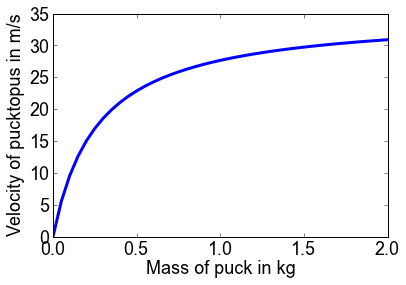# Defining new units¶

Sometimes we're dealing with a non-standard quantity, or we want to translate a real quantity to something we're using in our code (e.g., a mapping from real-world lengths to length in terms of pixels in a GUI application).

## Non-standard units example¶

Suppose we're part of the PyCon Canada organizing team. If every PyCon attendee drinks on average 200 mL of milk, how many bags of milk should we order?

To make a new unit, we use the UnitQuantity class.

In :
bags = pq.UnitQuantity('milk bags', pq.L * (4. / 3.))
person = pq.UnitQuantity('person', pq.dimensionless * 1)


This puts our new units (milk bags and persons) in terms of known units. A milk bag is 4/3 L, and a person is 1 thing that doesn't have a dimension.

We can then express our need in terms of milks bags and persons.

In :
need = 200 * (pq.mL / person)


If there are 250 PyCon attendees, then we would need to buy

In :
need *= 250 * person
print need

50000.0 mL

of milk. This is a big number; let's express it in litres instead.

In :
print need.rescale(pq.L)

50.0 L

Since we have defined how milk bags translate to litres, we can put this quantity in terms of milk bags instead.

In :
print need.rescale(bags)

37.5 milk bags

We can't purchase fractional bags. But since a Quantity is a subclass of ndarray, we can use NumPy's number manipulation capabilities to give us a clean answer.

In :
print np.ceil(need.rescale(bags))

38.0 milk bags

### Final words¶

quantities isn't perfect; (degrees stuff)

One last thing to say about quantities is a simple trick. If you ever find yourself needing the raw float value being represented, use Quantity.item().

In :
print need.item()
print need.rescale(bags).item()

50000.0
37.5

# A real-world example¶

These toy examples have hopefully convinced you that physical quantities are easy to use. But what about when you scale up to real scientific research code?

I originally started using quantities because it is used by Python-Neo, a package I use for neuroscientific data analysis. In my experience, I have had very few occasions where I had problems because of quantities, but many occasions where using quantities has improved my code significantly.

## Spike data¶

One of the types of data that neuroscientists collect is the times at which a neuron spikes.

We can generate some sham data (that doesn't match the statistics of real spike trains, but is good enough for illustrative purposes) with the following function.

In :
def generate_spikes(filename, neurons=20, time=20 * pq.s, event=11 * pq.s):
with open(filename, 'w') as f:
# Choose random rates
rates = np.random.randint(2, 10, size=neurons) * pq.Hz
for rate in rates:
# Randomly generate spikes
spikes = np.random.uniform(0, time, size=time * rate)
# Add spikes around the event
spikes = np.append(spikes, np.random.normal(event, size=rate * 5))
# Sort and save to text file
np.savetxt(f, (np.sort(spikes),), fmt="%f", delimiter=', ')

# Uncomment to call this function
# generate_spikes("spikes.csv")


We can then load that comma-separated value file into a list of numpy arrays with the following function.

Note that this cannot be loaded into a two-dimensional array because each individual spike train may be of different length.

In :
def load_spikes(filename):
with open(filename, 'r') as f:
return [np.array(map(float, line.split(','))) for line in lines]

spiketrains = [st * pq.s for st in load_spikes("spikes.csv")]


We can plot these spike trains using a scatterplot. This is called a spike raster plot.

In :
def raster_plot(spiketrains, event=None, window=None):
plt.clf()  # Start a fresh plot
if event is not None:
event.units = spiketrains.units
plt.axvline(event, lw=2, color='r')
plt.text(event, len(spiketrains) + 0.01 * len(spiketrains), "Event",
color='r', horizontalalignment='center', fontsize=12)

if window is not None:
window.units = spiketrains.units
plt.axvspan(event + window, event + window,
facecolor='#9696FF', zorder=0, ec='none')

lastspike = 0.0 * pq.s
firstspike = np.amin(spiketrains)
for j, st in enumerate(spiketrains):
lastspike = max(lastspike, np.amax(st))
firstspike = min(firstspike, np.amin(st))
plt.scatter(st, j * np.ones(st.shape), marker='|', color='k')
plt.ylim(-1, len(spiketrains))
plt.xlim(firstspike, lastspike)
plt.xlabel("Time in " + spiketrains.dimensionality.string)
plt.tight_layout()
plt.show()

raster_plot(spiketrains)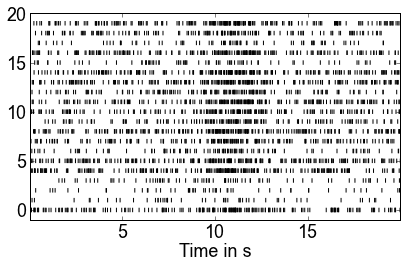Now that we have some spike trains, we want to do some data analysis.

## Peri-event rasters¶

While these spike trains are over a 20 second period, experimentally recorded spike trains can be extremely long -- it could be hours' worth of data.

We usually only want to do analysis around experimentally salient events. Let's suppose such an event happens 11 seconds into the recording time.

In :
event = 11 * pq.s
raster_plot(spiketrains, event=event)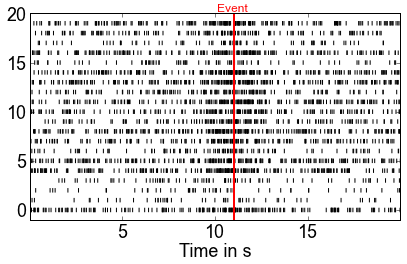Let's look at the spikes 0.5 s before the event, and 2 seconds after the event.

In :
window = (-0.5, 2) * pq.s
raster_plot(spiketrains, event=event, window=window)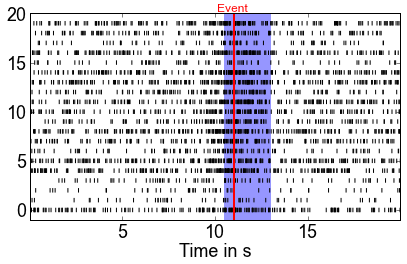In :
def time_slice(spikes, tstart, tend):
return spikes[np.logical_and(tstart <= spikes, spikes < tend)]

perievent = [time_slice(spikes, event + window, event + window)
for spikes in spiketrains]
raster_plot(perievent, event=event)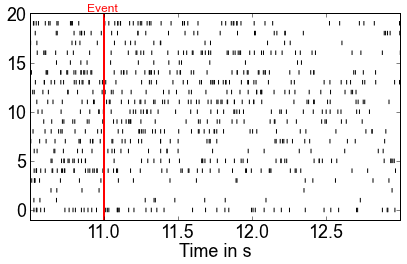We can do these same functions in terms of milliseconds without changing anything!

In :
event = 7000 * pq.ms
window = (-2500, 1000) * pq.ms
raster_plot(spiketrains, event=event, window=window)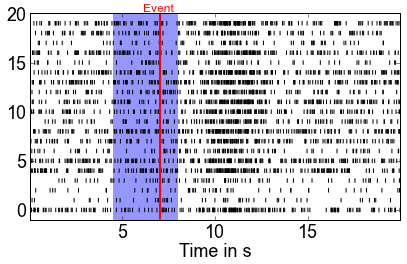We can even mix units!

In :
event = 5.0 * pq.s
window = (-1000, 1000) * pq.ms
raster_plot(spiketrains, event=event, window=window)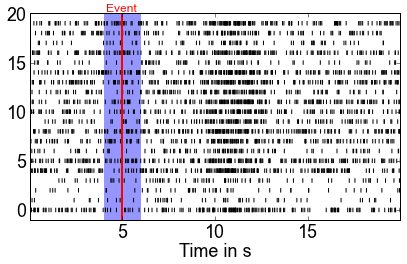## Spike binning¶

Sometimes in order to do calculations, we need to "bin" the spikes; that is, we discretize time into a finite set of bins, and sum up the number of spikes that occur within that time bin.

Often this binning requires confusing calculations in order to determine the number of bins in a time window. With quantities, however, this is easy.

In :
def bin_spikes(spikes, tstart, tend, binsize):
binsize.units = tstart.units
bins = np.arange(tstart, tend + binsize, binsize)
return np.histogram(spikes, bins=bins)

binsize = 20 * pq.ms
binned = np.vstack([bin_spikes(st, 0 * pq.s, 20 * pq.s, binsize) for st in spiketrains])


To plot the result, we can use pcolormesh to make an image in which the color of a cell corresponds to the number of spikes in that time bin.

In :
def binned_plot(bins, window=None):
plt.clf()
plt.pcolormesh(bins, cmap="gray_r")
if window is not None:
plt.axvspan(window, window, facecolor='b', alpha=0.5, ec='none')
plt.xlabel("Time bin")
plt.xlim(0, bins.shape)

# Discrete color bars take a bit of code
bounds = np.arange(0.5, np.amax(bins) + 1)
norm = mpl.colors.BoundaryNorm(bounds, len(bounds) - 1)
plt.tight_layout()
plt.colorbar(format="%d", spacing='proportional',
norm=norm, boundaries=bounds)

binned_plot(binned, (525, 650))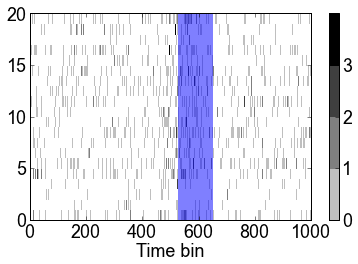It's easy with this code to bin only the perievent spikes.

In :
peribinned = np.vstack([bin_spikes(st, event + window,
event + window, binsize) for st in spiketrains])
binned_plot(peribinned)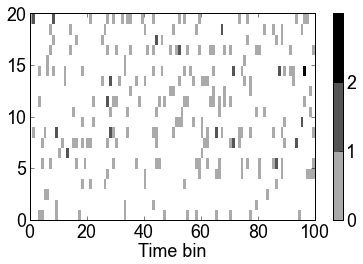Changing the bin size is easy, and we can use whichever units make the most sense.

In :
binsize = 50 * pq.ms
binned = np.vstack([bin_spikes(st, 0 * pq.s, 20 * pq.s, binsize) for st in spiketrains])
binwindow = (event + window).rescale(binsize.units) / binsize
binned_plot(binned, binwindow)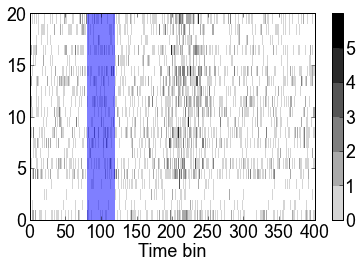In :
binsize = 0.05 * pq.s
binned = np.vstack([bin_spikes(st, 0 * pq.s, 20 * pq.s, binsize) for st in spiketrains])
binwindow = (event + window).rescale(binsize.units) / binsize
binned_plot(binned, binwindow)In [ ]: